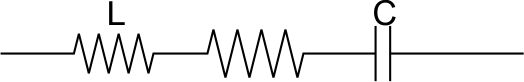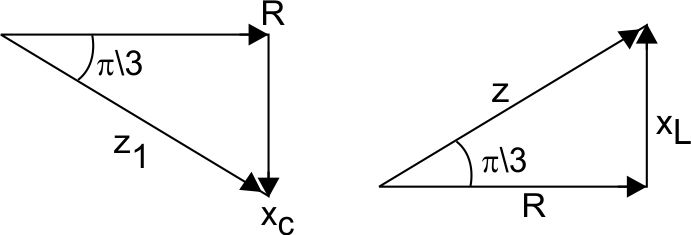# In an electrical circuit R, L, C and an a.c. voltage source are all connected in series. When L is removed from the circuit, the phase difference between the voltage and the current in the circuit is pi/3 . If instead, C is removed from the circuit, the phase difference is again pi/3 . The power factor of the circuit is:

As we learnt in

Impedence -

-

Power factor -

- wherein

resistance

impedencewhen C is removed from the Circuit

Power factor

Exams
Articles
Questions